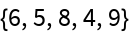#Function Repository Resource:

# DropSmallest

Drop the smallest values from a list

Contributed by: Sander Huisman
 ResourceFunction["DropSmallest"][list,n] drops the smallest n numbers from list. ResourceFunction["DropSmallest"][n] represents the operator form of DropSmallest.

## Examples

### Basic Examples

Drops the smallest two values:

 In:=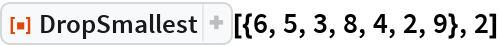Out=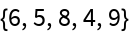Use the operator form by first creating an operator op:

 In:=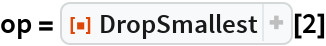Out=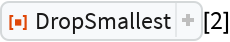Apply the operator:

 In:=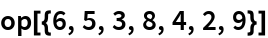Out=### Scope

With n=0, nothing is deleted:

 In:=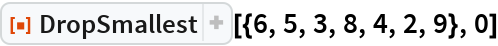Out=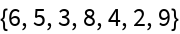A negative number deletes the largest n numbers:

 In:=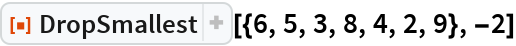Out=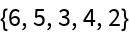### Properties and Relations

If n is larger than the length of the list then an empty list is returned:

 In:=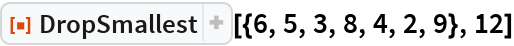Out=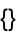DropSmallest is complementary to TakeSmallest:

 In:=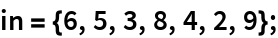In:=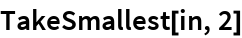Out=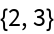In:=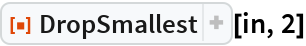Out=